# MOTION AND FORCES MOTION Motion A change in

• Slides: 20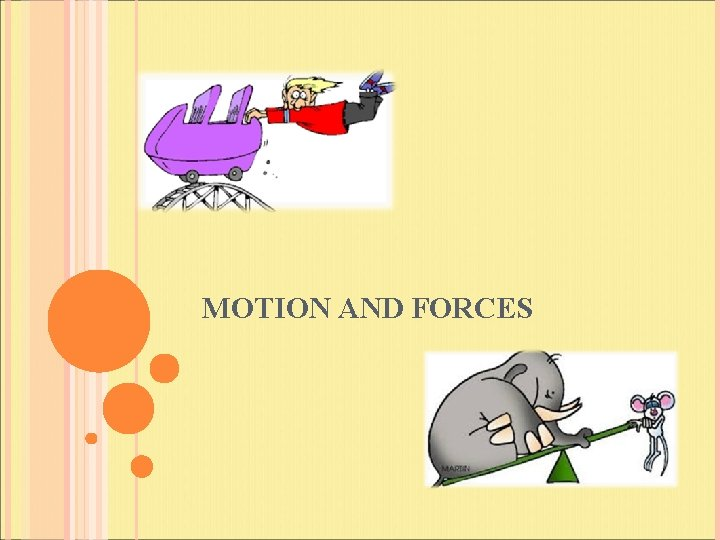MOTION AND FORCES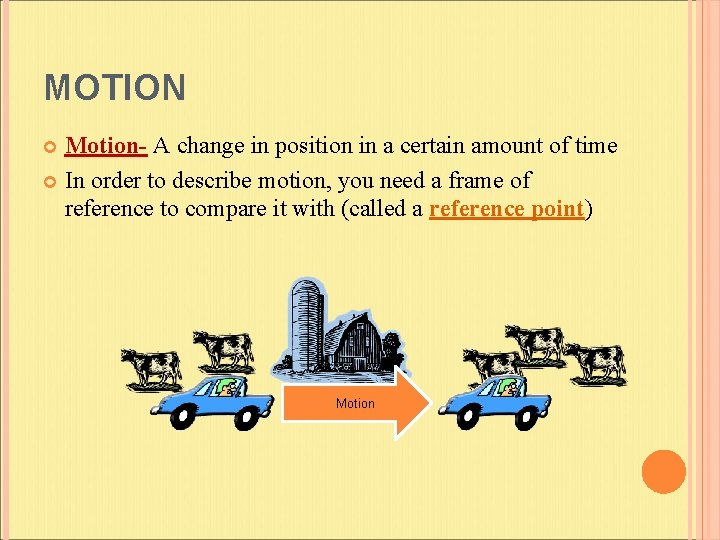MOTION Motion- A change in position in a certain amount of time In order to describe motion, you need a frame of reference to compare it with (called a reference point) MotionMOTION AND FORCES The motion of an object can change when one or more forces act on the object A force is a push or a pull (measured in Newtons (N) ) When one object pushes or pulls another object, the first object exerts a force on the second object A force is described by its strength and the direction in which it acts Arrows are used to show the direction and strength of forces Arrows points in the direction of the force Length of arrows indicate the strength of the force (the longer the arrow the stronger the force)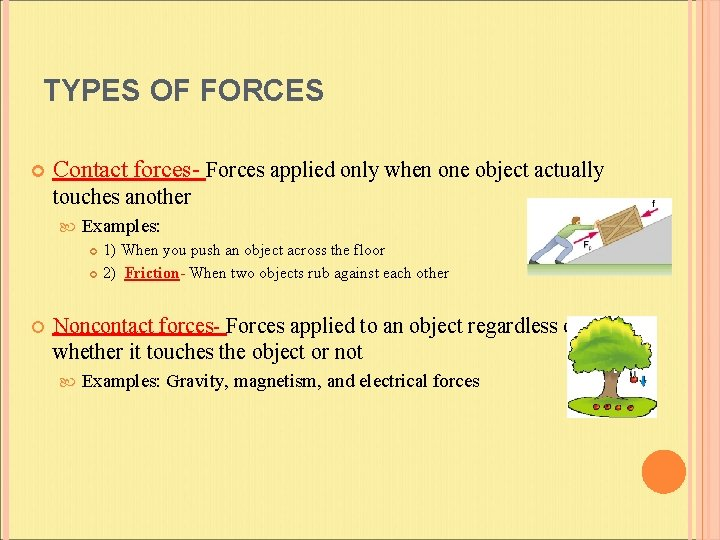TYPES OF FORCES Contact forces- Forces applied only when one object actually touches another Examples: 1) When you push an object across the floor 2) Friction- When two objects rub against each other Noncontact forces- Forces applied to an object regardless of whether it touches the object or not Examples: Gravity, magnetism, and electrical forces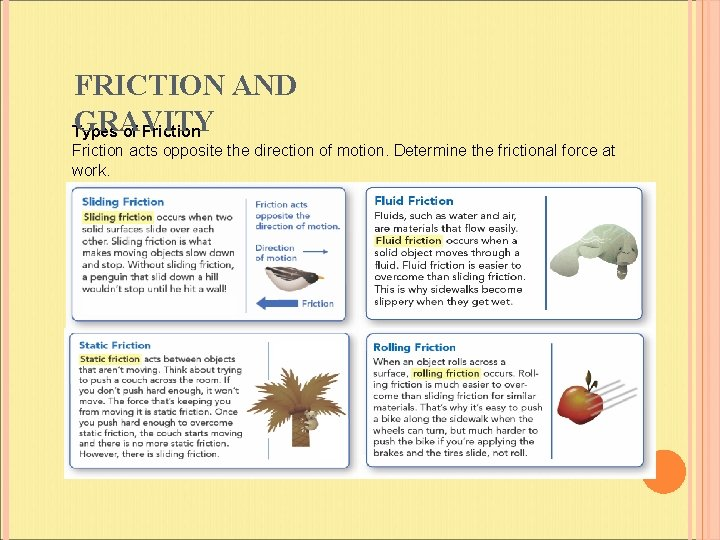FRICTION AND GRAVITY Types of Friction acts opposite the direction of motion. Determine the frictional force at work.FORCE AND GRAVITY Gravitational Attraction Gravitational attraction depends on two factors: mass and distance. Compare the gravitational force between different planets and their sun. -The greater the mass, the greater the force -The greater the distance, the lower the force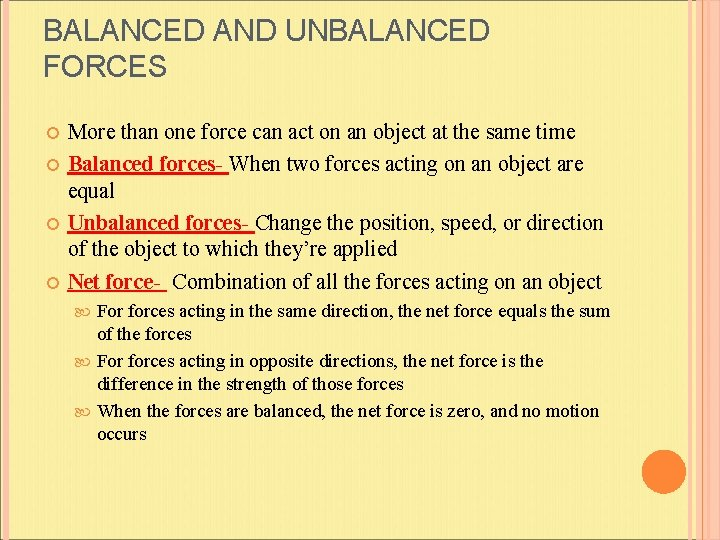BALANCED AND UNBALANCED FORCES More than one force can act on an object at the same time Balanced forces- When two forces acting on an object are equal Unbalanced forces- Change the position, speed, or direction of the object to which they’re applied Net force- Combination of all the forces acting on an object For forces acting in the same direction, the net force equals the sum of the forces For forces acting in opposite directions, the net force is the difference in the strength of those forces When the forces are balanced, the net force is zero, and no motion occurs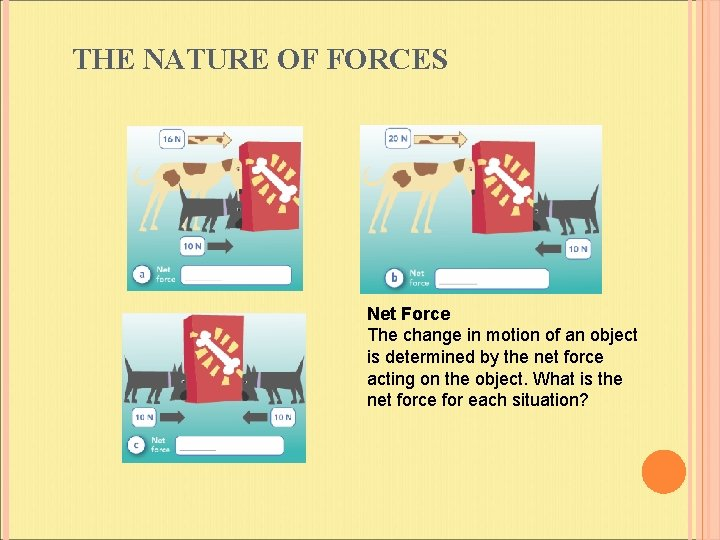THE NATURE OF FORCES Net Force The change in motion of an object is determined by the net force acting on the object. What is the net force for each situation?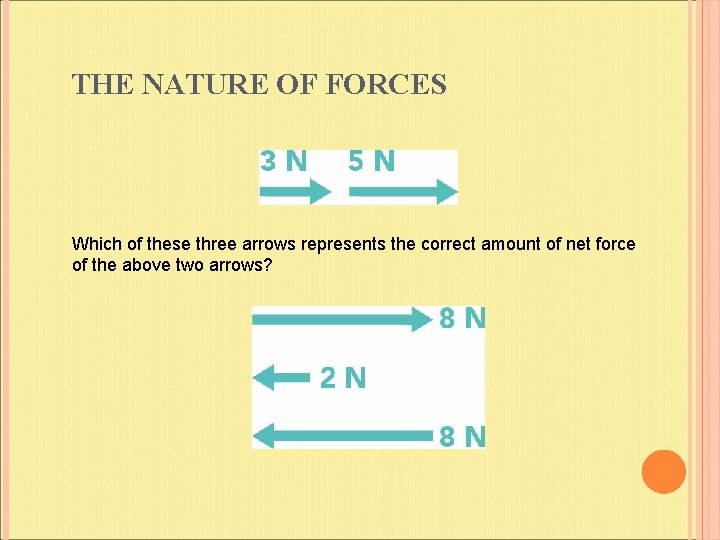THE NATURE OF FORCES Which of these three arrows represents the correct amount of net force of the above two arrows?SPEED Speed- The rate at which an object moves Speed = Distance Time Since distance is usually measured in meters and time in seconds, the unit for speed is given in meters per second (m/sec) Calculating speed from a graph The slope (the angle of the line on the graph) tells you the rate of change Slope = rise run Velocity- Speed in a given direction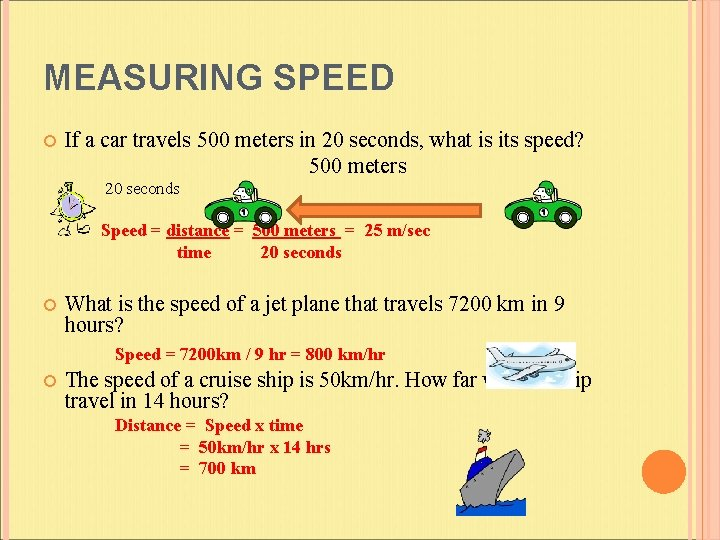MEASURING SPEED If a car travels 500 meters in 20 seconds, what is its speed? 500 meters 20 seconds Speed = distance = 500 meters = 25 m/sec time 20 seconds What is the speed of a jet plane that travels 7200 km in 9 hours? Speed = 7200 km / 9 hr = 800 km/hr The speed of a cruise ship is 50 km/hr. How far will the ship travel in 14 hours? Distance = Speed x time = 50 km/hr x 14 hrs = 700 kmACCELERATION Acceleration- The rate in change of velocity If something is accelerating, it is speeding up, slowing down, or changing direction The acceleration of an object is equal to its change in velocity divided by the time in which the change occurs Acceleration = Final velocity – Original Velocity Time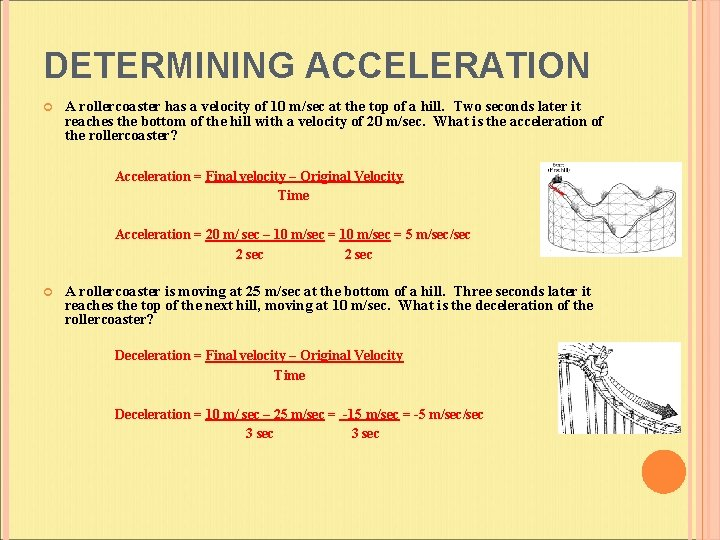DETERMINING ACCELERATION A rollercoaster has a velocity of 10 m/sec at the top of a hill. Two seconds later it reaches the bottom of the hill with a velocity of 20 m/sec. What is the acceleration of the rollercoaster? Acceleration = Final velocity – Original Velocity Time Acceleration = 20 m/ sec – 10 m/sec = 5 m/sec 2 sec A rollercoaster is moving at 25 m/sec at the bottom of a hill. Three seconds later it reaches the top of the next hill, moving at 10 m/sec. What is the deceleration of the rollercoaster? Deceleration = Final velocity – Original Velocity Time Deceleration = 10 m/ sec – 25 m/sec = -15 m/sec = -5 m/sec 3 secLAWS OF MOTION Newton’s laws of motion describe three states of motion Rest Constant motion Accelerated motion The laws also explain how forces cause all of the states of motion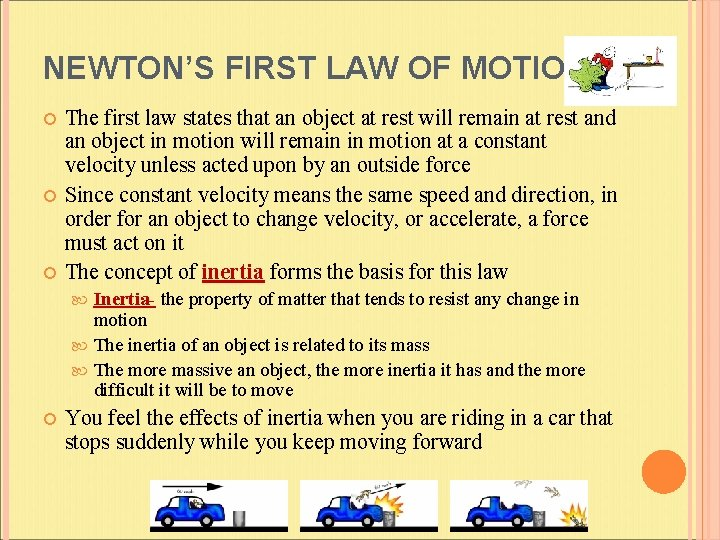NEWTON’S FIRST LAW OF MOTION The first law states that an object at rest will remain at rest and an object in motion will remain in motion at a constant velocity unless acted upon by an outside force Since constant velocity means the same speed and direction, in order for an object to change velocity, or accelerate, a force must act on it The concept of inertia forms the basis for this law Inertia- the property of matter that tends to resist any change in motion The inertia of an object is related to its mass The more massive an object, the more inertia it has and the more difficult it will be to move You feel the effects of inertia when you are riding in a car that stops suddenly while you keep moving forward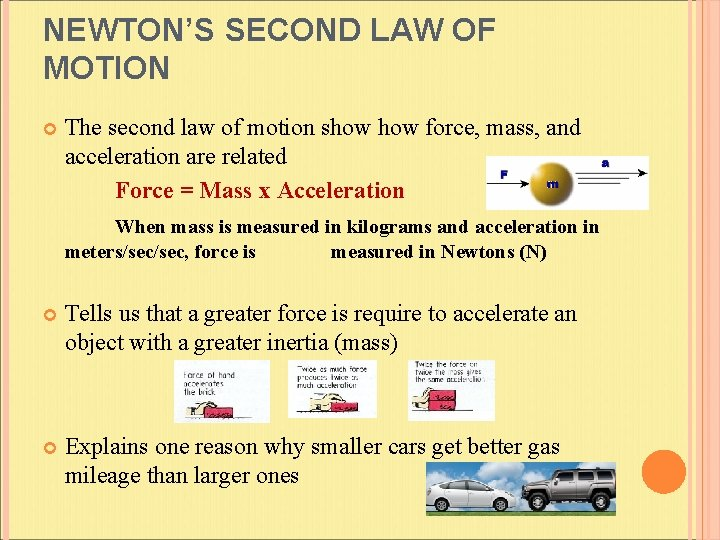NEWTON’S SECOND LAW OF MOTION The second law of motion show force, mass, and acceleration are related Force = Mass x Acceleration When mass is measured in kilograms and acceleration in meters/sec, force is measured in Newtons (N) Tells us that a greater force is require to accelerate an object with a greater inertia (mass) Explains one reason why smaller cars get better gas mileage than larger ones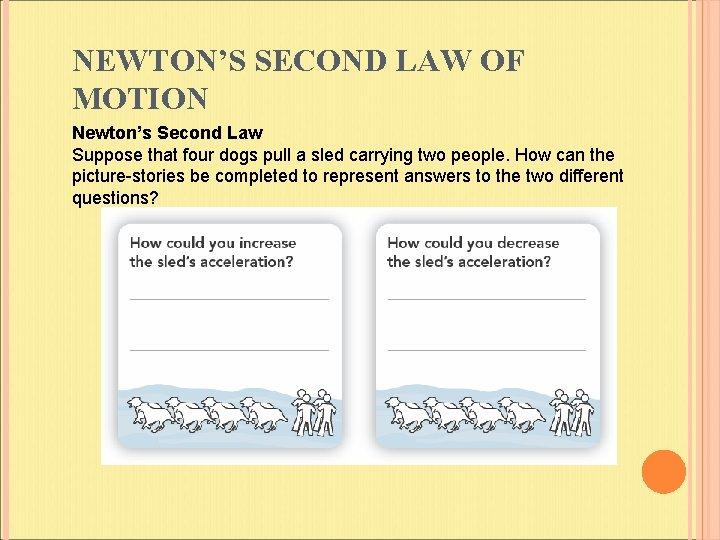NEWTON’S SECOND LAW OF MOTION Newton’s Second Law Suppose that four dogs pull a sled carrying two people. How can the picture-stories be completed to represent answers to the two different questions?NEWTON’S THIRD LAW OF MOTION States that for every action, there is an equal and opposite reaction According to the third law: Every force must have an equal and opposite force All forces come in pairs Action-reaction forces In every interaction, there is a pair of forces acting on the two interacting objects Action-reactions are not balanced (they are unbalanced) because they act on different objects Remember that unbalanced forces produce motion, but balanced forces do notNEWTON’S THIRD LAW IN MOTION You demonstrate third law when you walk Your feet push against the floor The floor pushes with an equal but opposite force against your feet You move forward The reaction engine utilizes the third law The thrust of the engine pushes against the Earth The Earth pushes with an equal but opposite force against the rocket Since it has less mass than the Earth, the rocket rises into the air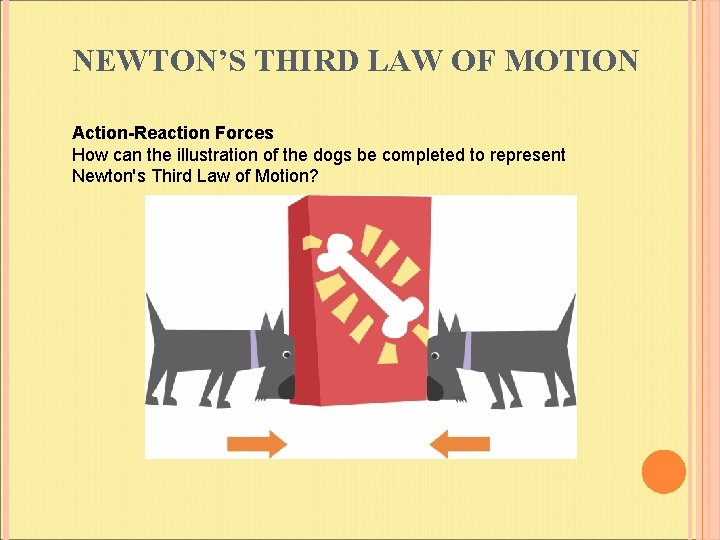NEWTON’S THIRD LAW OF MOTION Action-Reaction Forces How can the illustration of the dogs be completed to represent Newton's Third Law of Motion?